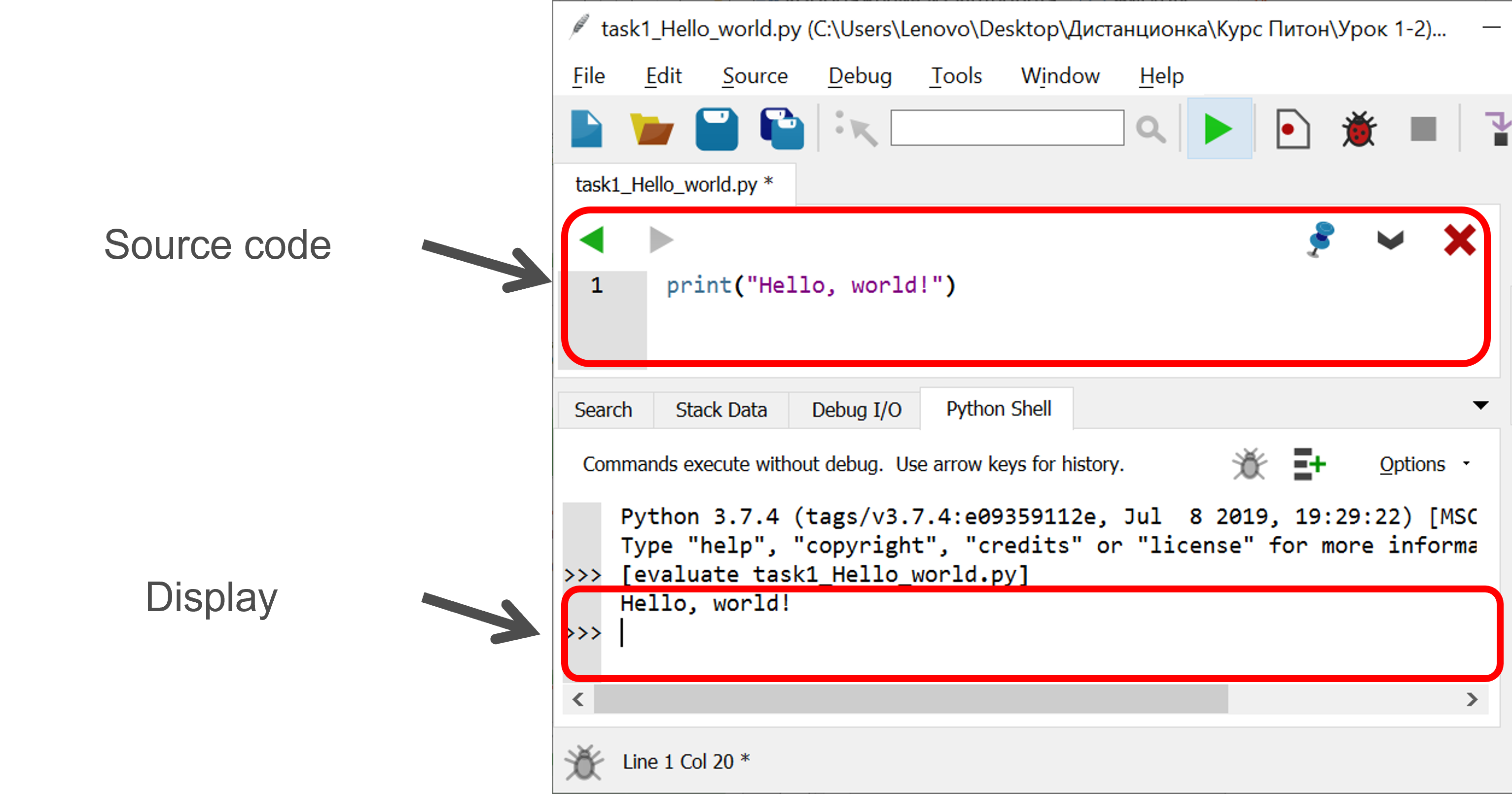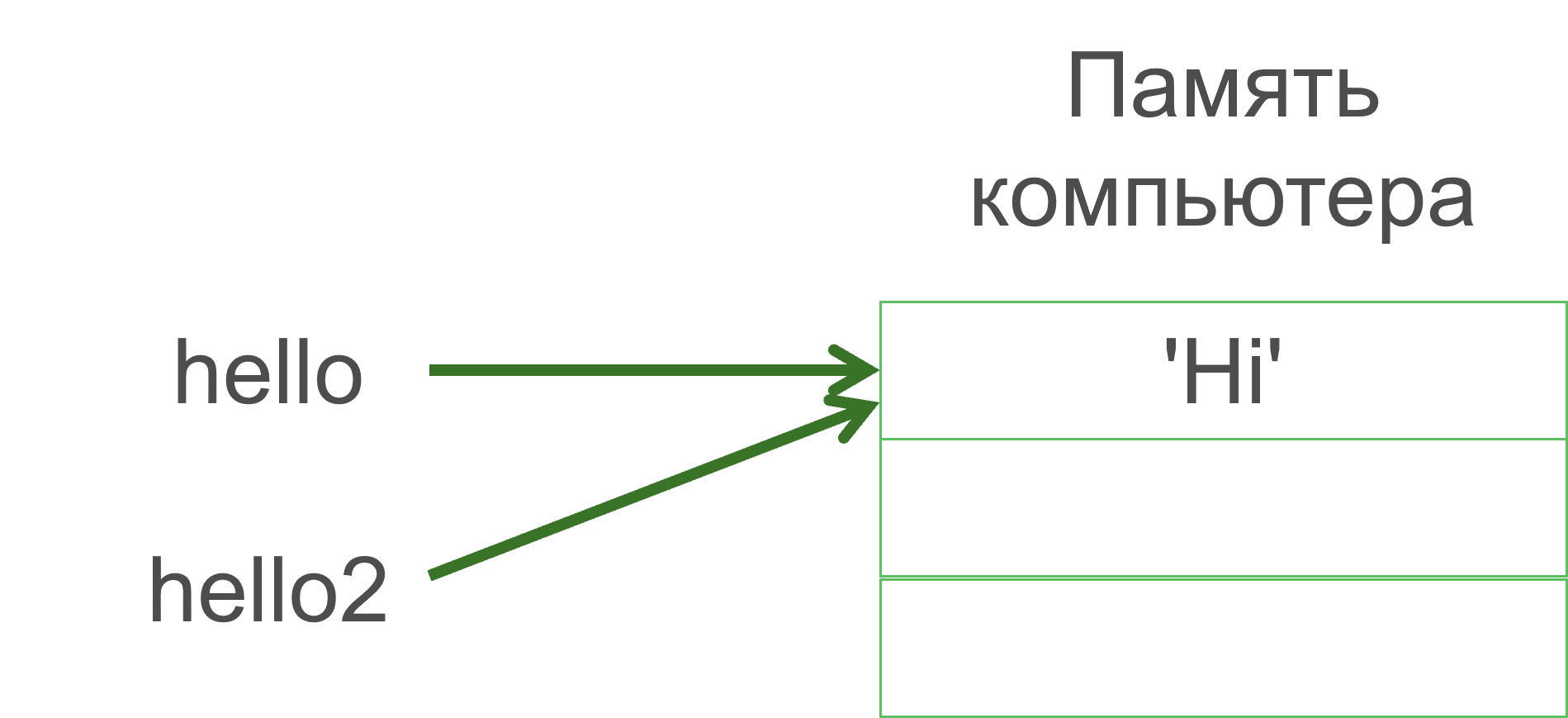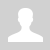Python. Output data (eng)

11.1.1.1 organize data output
11.1.1.2 use the escape sequences with data output

Output data / Вывод данных

Подписывайтесь на канал "Программирование на Python для чайников".

print(arguments) - function for output data on display.

Task. Output text "Hello, world!" on display:

`print("Hello, world!")`

Output data:

Hello, world!

Wing IDEWho is the best programmer?

```print("Hi!") print("I am the best programmer!")```

Output data:

Hi!
I am the best programmer!

Function print can have more than one argument:

`print(arg1, arg2, arg3, …)`

Arguments can be:

• Strings: “Hello”, “course of Python”, “” , …
• Variables: a, name, number5, …
• Math expressions: 4 * a, 20 / 7, …

Who is the best programmer? (use only one print)

`print("Hi!\nI am the best programmer!")`

Output data:

Hi!
I am the best programmer!

Escape sequences

 Escape sequences Display \\ \ \' ' \" " \n new line \t horizontal tab \u… 16-bit Unicode character in hexadecimal notation \U… 32-bit Unicode character in 32-ary notation \x… Hex value

Using escape sequences.

Example

`print("text1\ntext2")`

Display:

text1
text2

Example

`print("Carrot\t250 tenge\nBetroot\t280 tenge")`

Display:

Carrot      250 tenge
Betroot     280 tenge

Define the result of the next code

`print("\u1D66")`

Argument sep (separator)

Program:

`print("2", "+", "2", "=", "4", sep="")`

Display:

2+2=4

Program:

`print("a", "b", "c", "d", "e", sep="-")`

Display:

a-b-c-d-e

Argument end

What will output at the end of print

Program:

```print("1", end="")  print("23", end="-")  print("456") ```

Display:

123-456

Variables

A variable is an identifier that can change the stored value during the execution of the program.

```hello = 'Hi' hello2 = hello print(hello2)```Rules for creating variables in Python

• A variable name can only contain letters, digits, and underscores (A - z, 0-9, and _).
• Variable names are case sensitive (num, Num, and NUM are three different variables).
• Don't use reserved words (keywords) as a variable name.

```number = 10      # variable number of integer type assigns the value 10 pi = 3.14        # floating point pi is assigned the value 3.14 word = "Hello"   # variable word of string type assigns the value "Hello" print(number, pi, word)```

Variable can change the value in a program.

```word = "Astana" print("2017 - ", word) print("2018 –", word) word = "Nur-Sultan" print("2019 –", word)```

Output data:

2017 - Astana
2018 - Astana
2019 – Nur-Sultan

The comment starts with a hash character (#), and is followed by text that contains further explanations.

Example:

```# defining the post code postCode = 090014```

If we want to comment out several lines at once, then use """.

Example:

```"""comments comments comments"""```

Questions:

1. How to output data in a Python program?

2. Explain what is variable.

3. Describe rules for variable's name.

4. How can you use comments in the program?

Exercises:

Ex.1

Категория: Programming languages | Добавил: bzfar77 (02.09.2021)
Просмотров: 560 | Теги: Print, End, Sep, Output, variable | Рейтинг: 5.0/4
 Всего комментариев: 0